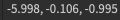# Inaccurate Values

I’ll keep this short.

I’m trying to make a light-switch system for my house, and it does work as intended, except for one measly thing.

When the switch’s X orientation value gets set to 6:

``````instance.CFrame.Orientation = Vector3.new(6,instance.CFrame.LookVector.Y,instance.CFrame.LookVector.Z)
``````

When I do this, it estimately sets to the number, but it has very small decimals randomly put into them:The actual value is meant to be `-6, 0, -1`.

If you know why, please tell me right now. Thank you.

3 Likes

It’s most likely a floating point error. The thing I am surprised about is how you’re assigning a CFrame’s orientation, shouldn’t this give an error?

2 Likes

Sorry, it’s actually `instance.Orientation`. No errors about that, it’s just the value being innacurate.

Also, what’s a floating point error?

1 Like

try math.round everything like this

``````instance.CFrame.Orientation = Vector3.new(math.round(6),math.round(instance.CFrame.LookVector.Y),math.round(instance.CFrame.LookVector.Z))
``````

and if you are curious why it prints decimal numbers, it is just common bugs which even shows on any type of program or computer systems (computer cant get natural number easily and get decimal instead)

2 Likes

Are you not able to round the whole vector, not the individual values, like this:

``````math.round(Vector3.new(x,y,z))
``````
2 Likes

you should check it to studio but in my opinion round cant be used to tables

2 Likes

Try to use `:PivotTo()`

``````:PivotTo(CFrame.new() * CFrame.Angles()) --U can use math.rad()
``````
2 Likes

The part I’m teleporting isn’t a model.

2 Likes

can you show me output of

``````
instance.CFrame.Orientation = Vector3.new(math.round(6),math.round(instance.CFrame.LookVector.Y),math.round(instance.CFrame.LookVector.Z))

``````
2 Likes

Can’t I just do this?

``````instance.Orientation += Vector3.new(math.round(6),0,0)
``````

It really just adds the X axis by 6, and there might be no decimals because of the `math.round()` you recommended, I don’t need to do this whole thing.

And I did check, and `CFrame.Orientation` doesn’t exist, and you can’t separately edit LookVector like that.

2 Likes

what is script you printed this decimal

1 Like

The part doesnt need to be a model.

1 Like

`:PivotTo()` is the same as changing the CFrame, it’s just used for models because you need to change more than one CFrame, but for parts it’s the exact same thing.

1 Like

I just recently found out why this happens.

It’s a common machine bug that can happen every time. They aren’t able to accurately set values.

To extend what you’ve just discovered, let’s just discuss it a bit more.

Developers are generally required to pass numbers at a fixed precision (double, float, int64, etc.). You could also adjust your numbers with arbitrary precision, but the current engine does not support those in order to provide all of the clients & devs of Roblox the same performance. Arbitrary precision may consume the entire memory. For instance, setting all components of a Vector3 is handled quickly with fewer digits of precision. If you were to apply thousands of significant digits, this may have resulted in memory consumption.